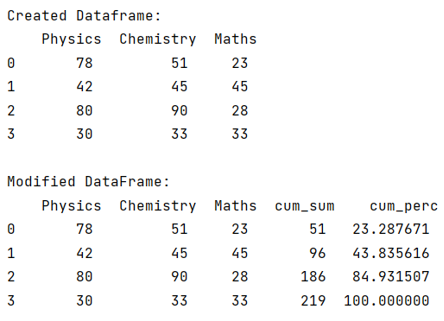# Python Pandas: Cumulative sum and percentage on column

Given a Pandas DataFrame, we need to get the cumulative sum and percentage on column.
Submitted by Pranit Sharma, on July 28, 2022

Pandas is a special tool that allows us to perform complex manipulations of data effectively and efficiently. Inside pandas, we mostly deal with a dataset in the form of DataFrame. DataFrames are 2-dimensional data structures in pandas. DataFrames consist of rows, columns, and data.

Pandas consist of almost every kind of mathematical and logical function which helps us to perform tough and long calculations with no effort.

To get the cumulative sum and percentage on the column, we will use df['col'].cumsum() method which will return an integer value.

For finding the percentage, we will divide the column in which we are storing the cumulative sum values by the column on which we want to operate.

At last, we will apply the sum() function to sum up all the values and then multiply all the values by 100.

Let us understand with the help of an example,

## Python code to get the cumulative sum and percentage on column

```# Importing Pandas package
import pandas as pd

# Creating a Dictionary
d = {
'Physics': [78, 42, 80, 30],
'Chemistry': [51, 45, 90, 33],
'Maths': [23, 45, 28, 33]
}

# Creating a dataframe
df = pd.DataFrame(d)

# Display Original dataframe
print("Created Dataframe:\n",df,"\n")

# Calculating cumulative sum
df['cum_sum'] = df['Chemistry'].cumsum()

# Calculating cumulative percentage
df['cum_perc'] =  100*df['cum_sum']/df['Chemistry'].sum()

# Display modified DataFrame
print("Modified DataFrame:\n",df)
```

Output:What's New

Top Interview Coding Problems/Challenges!

Languages: » C » C++ » C++ STL » Java » Data Structure » C#.Net » Android » Kotlin » SQL
Web Technologies: » PHP » Python » JavaScript » CSS » Ajax » Node.js » Web programming/HTML
Solved programs: » C » C++ » DS » Java » C#
Aptitude que. & ans.: » C » C++ » Java » DBMS
Interview que. & ans.: » C » Embedded C » Java » SEO » HR
CS Subjects: » CS Basics » O.S. » Networks » DBMS » Embedded Systems » Cloud Computing
» Machine learning » CS Organizations » Linux » DOS
More: » Articles » Puzzles » News/Updates